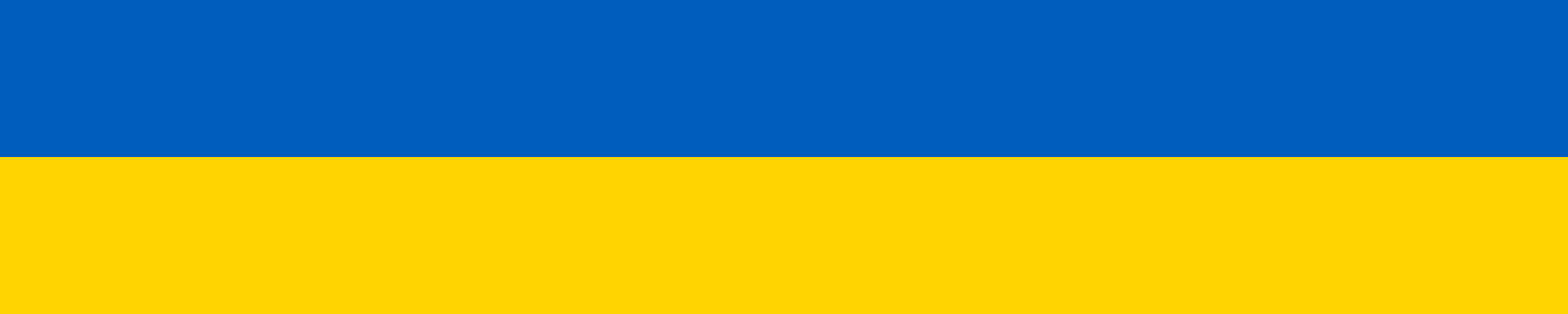# php if elseif else endif

Syntax with "endif" used mostly in complex templates where easier to find "endif" than "}":

```<?php
if( \$a > \$b ):
echo \$a.' is greater than '.\$b;
elseif( \$a < \$b ):
echo \$a.' is less than '.\$b;
else:
echo \$a.' equals '.\$b;
endif;
?>
```

Classic syntax:

```<?php
if( \$a > \$b ) {
echo \$a.' is greater than '.\$b;
} elseif( \$a < \$b ) {
echo \$a.' is less than '.\$b;
} else {
echo \$a.' equals '.\$b;
}
?>
```

### 1 comment on “php if elseif else endif”

1.ramesh

Thanks for this